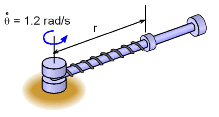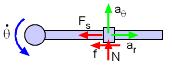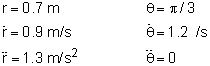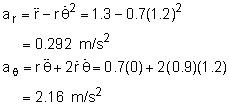Ch 2. Particle Force and Acceleration Multimedia Engineering Dynamics Rect.Coord. Normal/Tang. Coord. PolarCoord. Orbital Mechanics Computational Mechanics
 Chapter - Particle - 1. General Motion 2. Force & Accel. 3. Energy 4. Momentum - Rigid Body - 5. General Motion 6. Force & Accel. 7. Energy 8. Momentum 9. 3-D Motion 10. Vibrations Appendix Basic Math Units Basic Equations Sections Search eBooks Dynamics Fluids Math Mechanics Statics Thermodynamics Author(s): Kurt Gramoll ©Kurt GramollDYNAMICS - EXAMPLERotating Spring Arm Example A 5 kg collar slides along a rod that is rotating. The collar is also attached to the center with a spring. There is friction between the collar and the bar, μ = 0.3. At a particular time, dθ/dt = 1.2 rad/s, dr/dt = 0.9 m/s, d2r/dt2 = 1.3 m/s2, and r = 0.7 m. The angular velocity is constant. What is the force magnitude acting on the collar due to the spring? Neglect gravity. SolutionCollar Free-body Diagram with Accelerations The acceleration in the radial and theta directions need to be determined before summing forces. The given information is,Substituting into acceleration equations,Summing forces and accelerations give,      ΣF = maθ      N = 5 (2.16)      N = 10.8 N      ΣF = mar      -Fs - Ff = mar      -Fs - μN = 5 (0.292)      -Fs - 0.3 (10.8) = 1.46 Fs = -4.70 N or 4.70 N

Practice Homework and Test problems now available in the 'Eng Dynamics' mobile app
Includes over 400 problems with complete detailed solutions.
Available now at the Google Play Store and Apple App Store.